# AC 自动机

## 概述¶

AC 自动机是 以 Trie 的结构为基础，结合 KMP 的思想 建立的。

1. 基础的 Trie 结构：将所有的模式串构成一棵 Trie。
2. KMP 的思想：对 Trie 树上所有的结点构造失配指针。

## 字典树构建¶

AC 自动机在初始时会将若干个模式串丢到一个 Trie 里，然后在 Trie 上建立 AC 自动机。这个 Trie 就是普通的 Trie，该怎么建怎么建。

## 失配指针¶

AC 自动机利用一个 fail 指针来辅助多模式串的匹配。

1. 共同点：两者同样是在失配的时候用于跳转的指针。
2. 不同点：next 指针求的是最长 Border（即最长的相同前后缀），而 fail 指针指向所有模式串的前缀中匹配当前状态的最长后缀。

AC 自动机在做匹配时，同一位上可匹配多个模式串。

### 构建指针¶

1. 如果 $\text{trie}[\text{fail}[p],c]$ 存在：则让 u 的 fail 指针指向 $\text{trie}[\text{fail}[p],c]$。相当于在 $p$$\text{fail}[p]$ 后面加一个字符 c，分别对应 $u$$fail[u]$
2. 如果 $\text{trie}[\text{fail}[p],c]$ 不存在：那么我们继续找到 $\text{trie}[\text{fail}[\text{fail}[p]],c]$。重复 1 的判断过程，一直跳 fail 指针直到根结点。
3. 如果真的没有，就让 fail 指针指向根结点。

### 例子¶

1. 黄色结点：当前的结点 $u$
2. 绿色结点：表示已经 BFS 遍历完毕的结点，
3. 橙色的边：fail 指针。
4. 红色的边：当前求出的 fail 指针。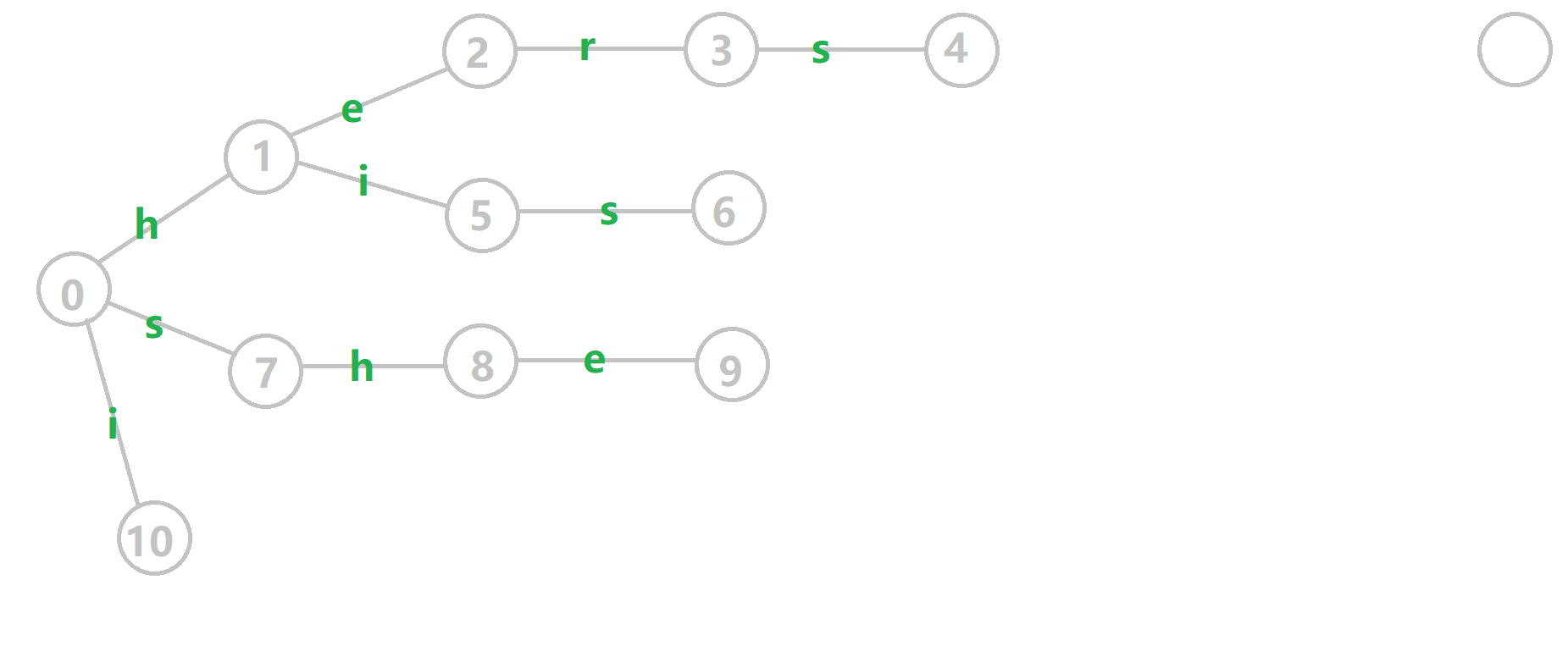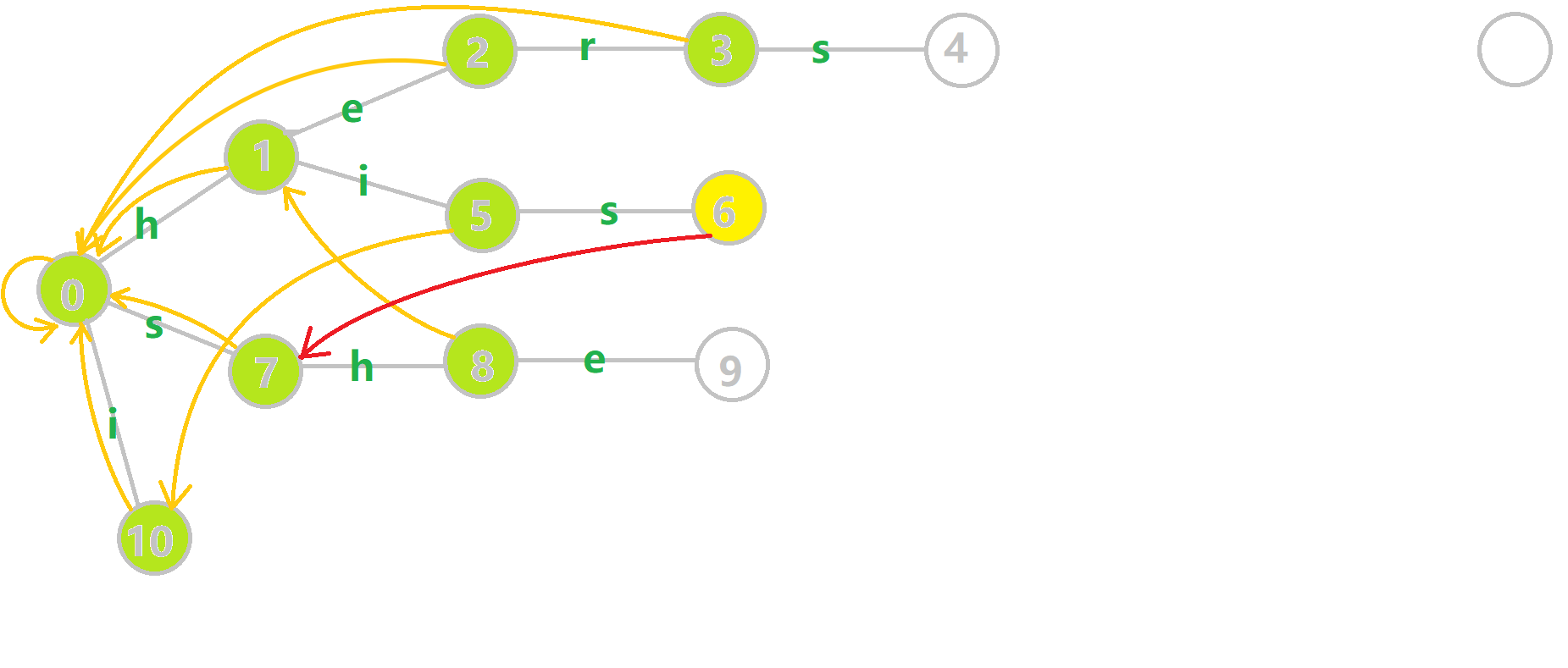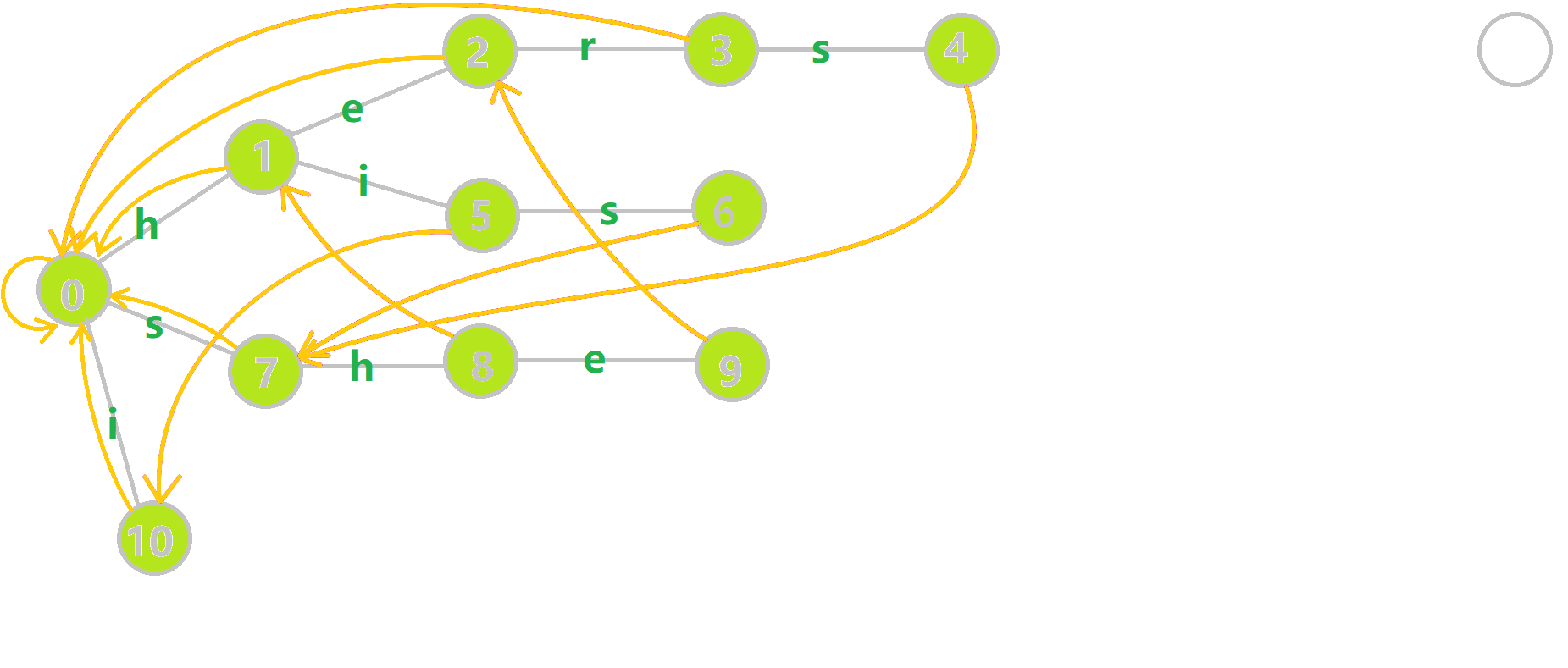## 字典树与字典图¶

1. tr[u,c]：有两种理解方式。我们可以简单理解为字典树上的一条边，即 $\text{trie}[u,c]$；也可以理解为从状态（结点）$u$ 后加一个字符 c 到达的状态（结点），即一个状态转移函数 $\text{trans}(u,c)$。下文中我们将用第二种理解方式继续讲解。
2. 队列 q：用于 BFS 遍历字典树。
3. fail[u]：结点 $u$ 的 fail 指针。
  1 2 3 4 5 6 7 8 9 10 11 12 13 14 15 // C++ Version void build() { for (int i = 0; i < 26; i++) if (tr[i]) q.push(tr[i]); while (q.size()) { int u = q.front(); q.pop(); for (int i = 0; i < 26; i++) { if (tr[u][i]) fail[tr[u][i]] = tr[fail[u]][i], q.push(tr[u][i]); else tr[u][i] = tr[fail[u]][i]; } } } 
  1 2 3 4 5 6 7 8 9 10 11 12 13 14 # Python Version def build(): for i in range(0, 26): if tr[i] == 1: q.append(tr[i]) while len(q) > 0: u = q q.pop() for i in range(0, 26): if tr[u][i] == 1: fail[tr[u][i]] = tr[fail[u]][i] q.append(tr[u][i]) else: tr[u][i] = tr[fail[u]][i] 

1. 如果 $\text{trans}[u][i]$ 存在，我们就将 $\text{trans}[u][i]$ 的 fail 指针赋值为 $\text{trans}[\text{fail}[u]][i]$。这里似乎有一个问题。根据之前的讲解，我们应该用 while 循环，不停的跳 fail 指针，判断是否存在字符 i 对应的结点，然后赋值，但是这里通过特殊处理简化了这些代码。
2. 否则，令 $\text{trans}[u][i]$ 指向 $\text{trans}[\text{fail}[u]][i]$ 的状态。

$\text{trans}[S][c]$ 相当于是在 $S$ 后添加一个字符 c 变成另一个状态 $S'$。如果 $S'$ 存在，说明存在一个模式串的前缀是 $S'$，否则我们让 $\text{trans}[S][c]$ 指向 $\text{trans}[\text{fail}[S]][c]$。由于 $\text{fail}[S]$ 对应的字符串是 $S$ 的后缀，因此 $\text{trans}[\text{fail}[S]][c]$ 对应的字符串也是 $S'$ 的后缀。

tr 数组还有另一种比较简单的理解方式：如果在位置 $u$ 失配，我们会跳转到 $\text{fail}[u]$ 的位置。所以我们可能沿着 fail 数组跳转多次才能来到下一个能匹配的位置。所以我们可以用 tr 数组直接记录记录下一个能匹配的位置，这样就能节省下很多时间。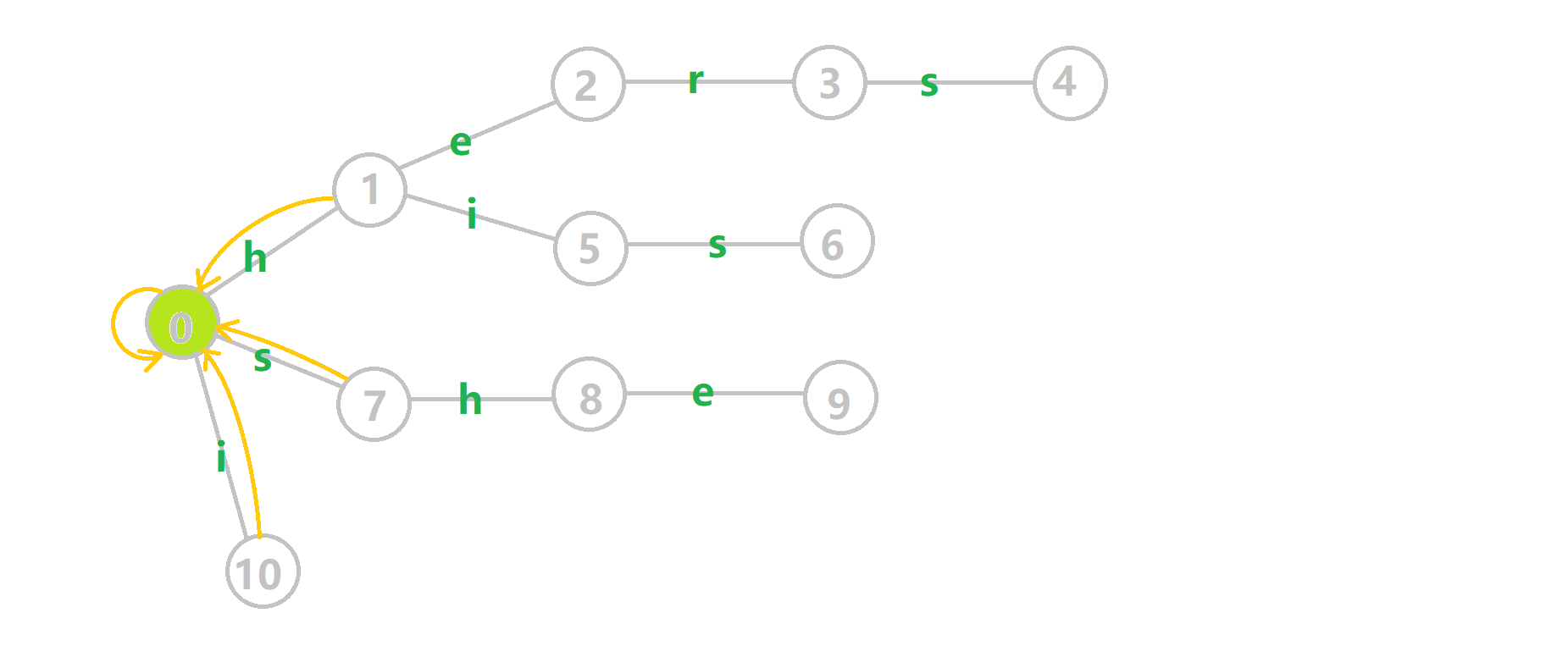1. 蓝色结点：BFS 遍历到的结点 u
2. 蓝色的边：当前结点下，AC 自动机修改字典树结构连出的边。
3. 黑色的边：AC 自动机修改字典树结构连出的边。
4. 红色的边：当前结点求出的 fail 指针
5. 黄色的边：fail 指针
6. 灰色的边：字典树的边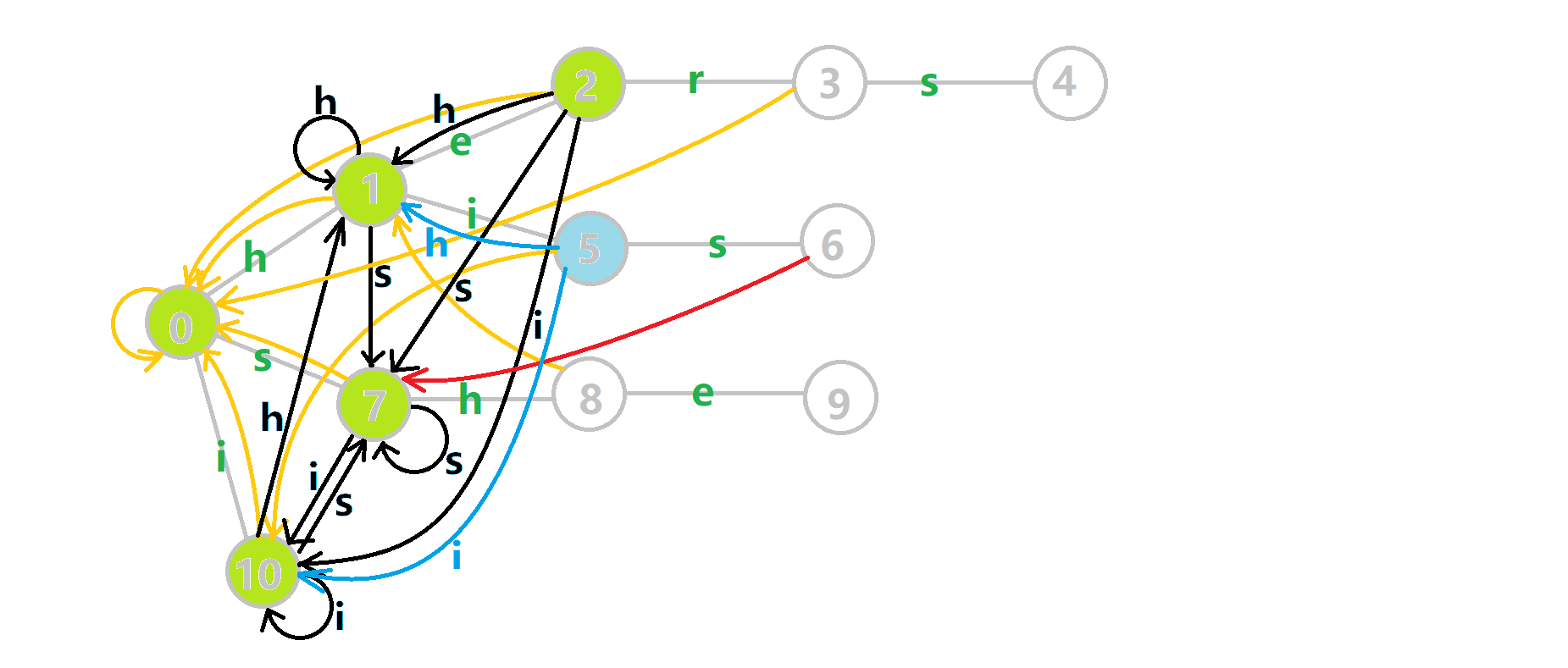## 多模式匹配¶

  1 2 3 4 5 6 7 8 9 10 11 // C++ Version int query(char *t) { int u = 0, res = 0; for (int i = 1; t[i]; i++) { u = tr[u][t[i] - 'a']; // 转移 for (int j = u; j && e[j] != -1; j = fail[j]) { res += e[j], e[j] = -1; } } return res; } 
  1 2 3 4 5 6 7 8 9 10 11 12 13 # Python Version def query(t): u, res = 0, 0 i = 1 while t[i] == False: u = tr[u][t[i] - ord('a')] j = u while j == True and e[j] != -1: res += e[j] e[j] = -1 j = fail[j] i += 1 return res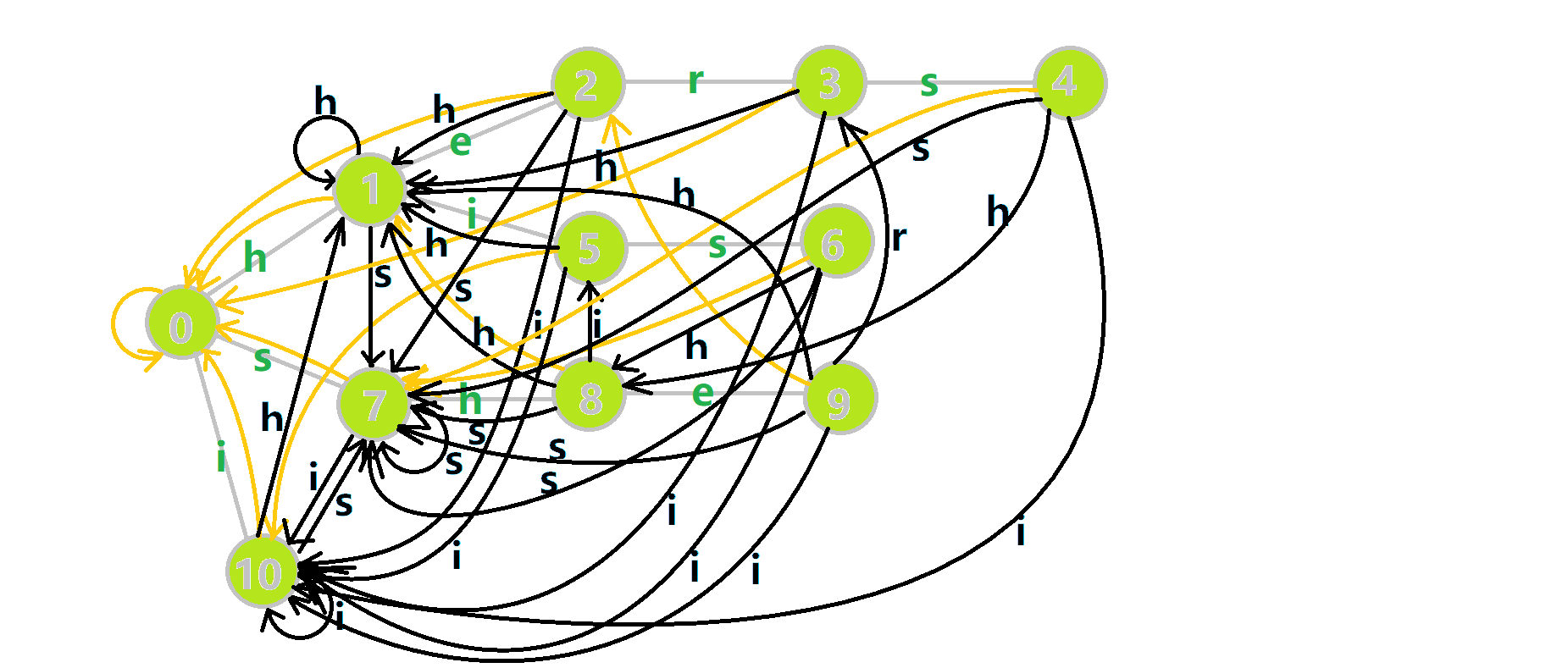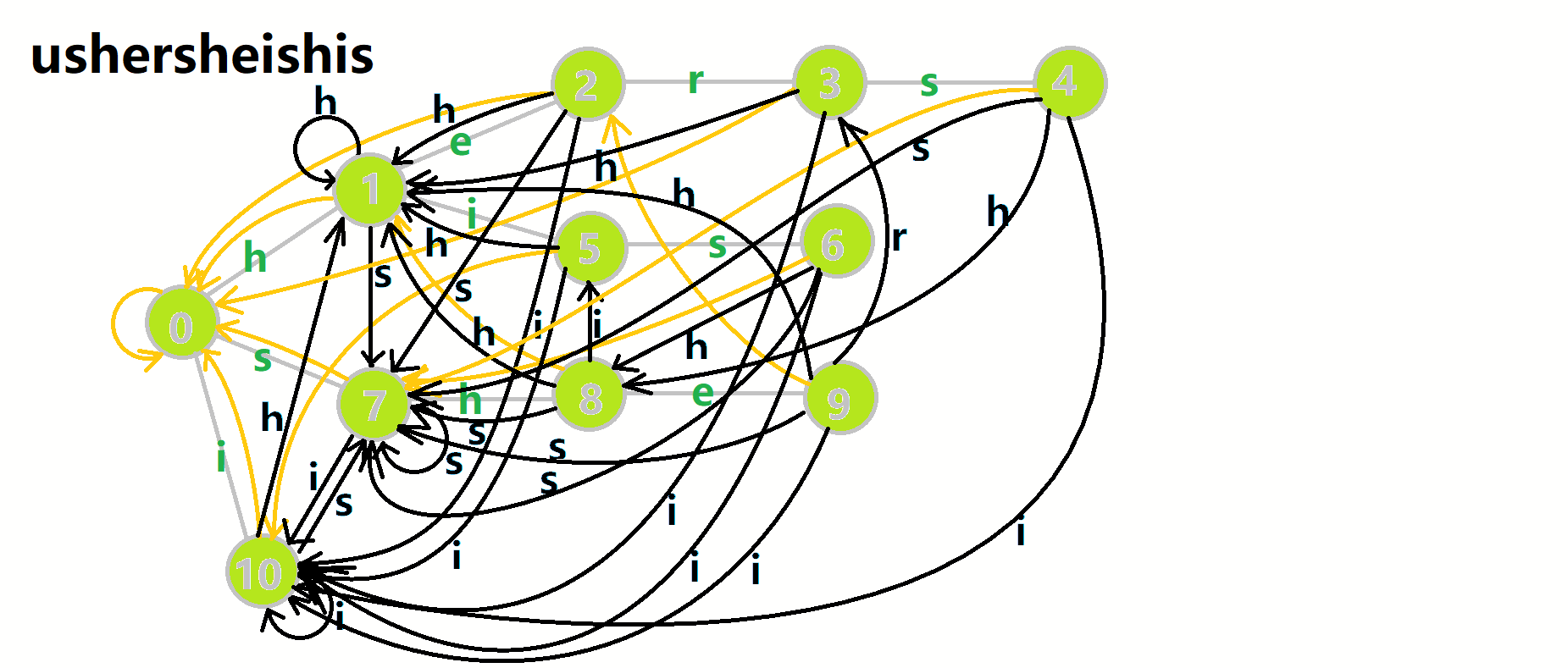1. 红色结点：$p$ 结点
2. 粉色箭头：$p$ 在自动机上的跳转，
3. 蓝色的边：成功匹配的模式串
4. 蓝色结点：示跳 fail 指针时的结点（状态）。

## 总结¶

LuoguP3808【模板】AC 自动机（简单版）

  1 2 3 4 5 6 7 8 9 10 11 12 13 14 15 16 17 18 19 20 21 22 23 24 25 26 27 28 29 30 31 32 33 34 35 36 37 38 39 40 41 42 43 44 45 46 47 48 49 50 51 52 53 54 55 56 57 58 59 #include using namespace std; const int N = 1e6 + 6; int n; namespace AC { int tr[N], tot; int e[N], fail[N]; void insert(char *s) { int u = 0; for (int i = 1; s[i]; i++) { if (!tr[u][s[i] - 'a']) tr[u][s[i] - 'a'] = ++tot; // 如果没有则插入新节点 u = tr[u][s[i] - 'a']; // 搜索下一个节点 } e[u]++; // 尾为节点 u 的串的个数 } queue q; void build() { for (int i = 0; i < 26; i++) if (tr[i]) q.push(tr[i]); while (q.size()) { int u = q.front(); q.pop(); for (int i = 0; i < 26; i++) { if (tr[u][i]) { fail[tr[u][i]] = tr[fail[u]][i]; // fail数组：同一字符可以匹配的其他位置 q.push(tr[u][i]); } else tr[u][i] = tr[fail[u]][i]; } } } int query(char *t) { int u = 0, res = 0; for (int i = 1; t[i]; i++) { u = tr[u][t[i] - 'a']; // 转移 for (int j = u; j && e[j] != -1; j = fail[j]) { res += e[j], e[j] = -1; } } return res; } } // namespace AC char s[N]; int main() { scanf("%d", &n); for (int i = 1; i <= n; i++) scanf("%s", s + 1), AC::insert(s); scanf("%s", s + 1); AC::build(); printf("%d", AC::query(s)); return 0; } 

P3796【模板】AC 自动机（加强版）

  1 2 3 4 5 6 7 8 9 10 11 12 13 14 15 16 17 18 19 20 21 22 23 24 25 26 27 28 29 30 31 32 33 34 35 36 37 38 39 40 41 42 43 44 45 46 47 48 49 50 51 52 53 54 55 56 57 58 59 60 61 62 63 64 65 66 67 68 69 70 71 72 73 74 75 76 77 #include using namespace std; const int N = 156, L = 1e6 + 6; namespace AC { const int SZ = N * 80; int tot, tr[SZ]; int fail[SZ], idx[SZ], val[SZ]; int cnt[N]; // 记录第 i 个字符串的出现次数 void init() { memset(fail, 0, sizeof(fail)); memset(tr, 0, sizeof(tr)); memset(val, 0, sizeof(val)); memset(cnt, 0, sizeof(cnt)); memset(idx, 0, sizeof(idx)); tot = 0; } void insert(char *s, int id) { // id 表示原始字符串的编号 int u = 0; for (int i = 1; s[i]; i++) { if (!tr[u][s[i] - 'a']) tr[u][s[i] - 'a'] = ++tot; u = tr[u][s[i] - 'a']; // 转移 } idx[u] = id; // 以 u 为结尾的字符串编号为 idx[u] } queue q; void build() { for (int i = 0; i < 26; i++) if (tr[i]) q.push(tr[i]); while (q.size()) { int u = q.front(); q.pop(); for (int i = 0; i < 26; i++) { if (tr[u][i]) { fail[tr[u][i]] = tr[fail[u]][i]; // fail数组：同一字符可以匹配的其他位置 q.push(tr[u][i]); } else tr[u][i] = tr[fail[u]][i]; } } } int query(char *t) { // 返回最大的出现次数 int u = 0, res = 0; for (int i = 1; t[i]; i++) { u = tr[u][t[i] - 'a']; for (int j = u; j; j = fail[j]) val[j]++; } for (int i = 0; i <= tot; i++) if (idx[i]) res = max(res, val[i]), cnt[idx[i]] = val[i]; return res; } } // namespace AC int n; char s[N], t[L]; int main() { while (~scanf("%d", &n)) { if (n == 0) break; AC::init(); // 数组清零 for (int i = 1; i <= n; i++) scanf("%s", s[i] + 1), AC::insert(s[i], i); // 需要记录该字符串的序号 AC::build(); scanf("%s", t + 1); int x = AC::query(t); printf("%d\n", x); for (int i = 1; i <= n; i++) if (AC::cnt[i] == x) printf("%s\n", s[i] + 1); } return 0; } 

## 拓展¶

### 确定有限状态自动机¶

1. 状态集合 $Q$
2. 字符集 $\Sigma$
3. 状态转移函数 $\delta:Q\times \Sigma \to Q$，即 $\delta(q,\sigma)=q',\ q,q'\in Q,\sigma\in \Sigma$
4. 一个开始状态 $s\in Q$
5. 一个接收的状态集合 $F\subseteq Q$

### KMP 自动机¶

KMP 自动机就是一个不断读入待匹配串，每次匹配时走到接受状态的 DFA。如果共有 $m$ 个状态，第 $i$ 个状态表示已经匹配了前 $i$ 个字符。那么我们定义 $\text{trans}_{i,c}$ 表示状态 $i$ 读入字符 $c$ 后到达的状态，$\text{next}_{i}$ 表示 prefix function，则有：

trans_{i,c} = \begin{cases} i + 1, & \text{if }b_{i} = c \\[2ex] \text{trans}_{next_{i},c}, & \text{else} \end{cases}

（约定 $\text{next}_{0}=0$